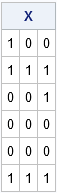I recently needed to solve a fun programming problem. I challenge other SAS programmers to solve it, too! The problem is easy to state: Given a long sequence of digits, can you write a program to count how many times a particular subsequence occurs? For example, if I give you a sequence of 1,000 digits, can you determine whether the five-digit pattern {1 2 3 4 5} appears somewhere as a subsequence? How many times does it appear?

If the sequence is stored in a data set with one digit in each row, then SAS DATA step programmers might suspect that the LAG function will be useful for solving this problem. The LAG function enables a DATA step to examine the values of several digits simultaneously.

The SAS/IML language also has a LAG function which enables you to form a matrix of lagged values. This leads to an interesting way use vectorized computations to solve this problem. The following SAS/IML program defines a small eight-digit set of numbers in which the pattern {1 2 3} appears twice. The LAG function in SAS/IML accepts a vector of lags and creates a matrix where each column is a lagged version of the input sequence:

```/* Find a certain pattern in sequence of digits */ proc iml; Digit = {1,1,2,3,3,1,2,3}; /* digits to search */ target = {1 2 3}; /* the pattern to find */ p = ncol(target); /* length of target sequence */ D = lag(Digit, (p-1):0); /* columns shift the digits */ print D;```The output shows a three-column matrix (D) that contains the second, first, and zeroth lag (in that order) for the input sequence. Notice that if I am searching for a particular three-digit pattern, this matrix is very useful. The rows of this matrix are all three-digit patterns that appear in the original sequence. Consequently, to search for a three-digit pattern, I can use the rows of the matrix D.

To make the task easier, you can delete the first two rows, which contain missing values. You can also form a binary matrix X that has the value X[i,j]=1 when the j_th element of the pattern equals the j_th element of the i_th row, and 0 otherwise, as shown in the following:

```D = D[p:nrow(Digit),]; /* delete first p rows */ X = (D=target); /* binary matrix */ print X;```Notice that in SAS/IML, the comparison operator (=) can perform a vector comparison. The binary comparison operator detects that the matrix on the left (D) and the vector on the right (target) both contain three columns. Therefore the operator creates the three-column logical matrix X, as shown. The X matrix has a wonderful property: a row of X contains all 1s if and only if the corresponding row of D matches the target pattern. So to find matches, you just need to sum the values in the rows of X. If the row sum equals the number of digits in the pattern, then that row indicates a place where the target pattern appears in the original sequence.

You can program this test in PROC IML as follows. The subscript reduction operator [,+] is shorthand for "compute the sum of each row over all columns".

```/* sum across columns. Which rows contain all 1s? */ b = (X[,+] = p); /* b[i]=1 if i_th row matches target */ NumRepl = sum(b); /* how many times does target appear? */ if NumRepl=0 then print "The target does not appear in the digits"; else print "The target appears at location " (loc(b)), /* print 1st location */ "The target appears" (NumRepl) "times.";```The program discovered that the target pattern appears in the sequence twice. The first appearance begins with the second digit in the sequence. The pattern also appears in the sequence at the sixth position, although that information is not printed.

Notice that you can solve this problem in SAS/IML without writing any loops. Instead, you can use the LAG function to convert the N-digit sequence into a matrix with N-p rows and p columns. You can then test whether the target pattern matches one of the rows.

### Your turn! Can you solve this problem?

Now that I have shown one way to solve the problem, I invite SAS programmers to write their own program to determine whether a specified pattern appears in a numeric sequence. You can use the DATA step, DS2, SQL, or any other SAS language.

Post a comment to submit your best SAS program. Extra points for programs that count all occurrences of the pattern and display the location of the first occurrence.

To help you debug your program, here is test data that we can all use. It contains 10 million random digits:

```data Have(keep=Digit); call streaminit(31488); do i = 1 to 1e7; Digit = floor(10*rand("uniform")); output; end; run;```

To help you determine if your program is correct, you can use the following results. In this sequence of digits:

• The five-digit pattern {1 2 3 4 5} occurs 101 times and the first appearance begins at row 34417
• The six-digit patter {6 5 4 3 2 1} occurs 15 times and the first appearance begins at row 120920

You can verify these facts by using PROC PRINT as follows:

```proc print data=Have(firstobs=34417 obs=34421); run; proc print data=Have(firstobs=120920 obs=120925); run;```

Happy programming!

The post Find a pattern in a sequence of digits appeared first on The DO Loop.